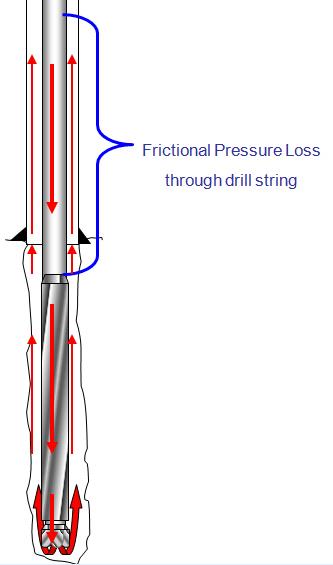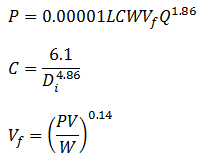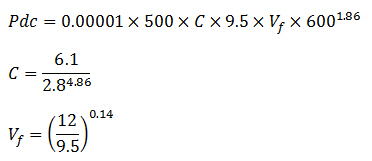# Pressure Losses Through Drill String CalculationTo determine pressure drop while the drilling mud is passing through a circular pipe (drill pipe and drill collar), you can calculate pressure loss with the following equation:Assumptions:

Turbulent viscosity = 3 cp

Friction factor = 0.378(Q/D)-0.14

The first equation has a coefficient (C) to take into account the friction and the geometry of the bore ID.

Where:

P = pressure loss, psi

L = length of pipe, ft

W = mud density, ppg

Vf = viscosity correction factor

PV = plastic viscosity, centipoises

Q = flow rate, gpm

Di = inside diameter of pipe, inch

C = general coefficient for internal diameter of pipe

Determine the pressure loss in the drill string based on the following information

Drill pipe length = 5000 ft

ID of drill pipe = 3.34 inch

Drill collar length = 500 ft

ID of drill collar = 2.8 inch

Mud Weight = 9.5 ppg

Plastic Viscosity = 12 centipoise

Flow rate = 600 gpm

Pressure loss in the drill string composes of two parts which are pressure loss in drill pipe and drill collar.

We can write the relationship like this:

Pt = Pdp + Pdc

Where; Pt = total pressure loss in drill string

Pdp = pressure loss in drill pipe

Pdc = pressure loss in drill collar

Determine pressure loss in drill pipe with following steps:Pdp = 1254

Determine pressure loss in drill collar with following steps:Pdc = 295

Pt = 1254 + 295 = 1,549 psi

Total pressure loss in the drill string equates to 1,549 psi.

Ref books:

Lapeyrouse, N.J., 2002. Formulas and calculations for drilling, production and workover, Boston: Gulf Professional publishing.

Bourgoyne, A.J.T., Chenevert , M.E. & Millheim, K.K., 1986. SPE Textbook Series, Volume 2: Applied Drilling Engineering, Society of Petroleum Engineers.

Mitchell, R.F., Miska, S. & Aadny, B.S., 2011. Fundamentals of drilling engineering, Richardson, TX: Society of Petroleum Engineers.

Share the joyWorking in the oil field and loving to share knowledge.

### 3 Responses to Pressure Losses Through Drill String Calculation

1.Luis says:

Hi,
The above formula is valid for all units?, for example the MW in sg and length of DP in meter, can we still use the above formula to calculate the pressure loss in drill pipe?

Thanks.
Luis

2.engineer says:

i think this advance step i need the first step
because i’m training